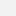# Humboldt-Universität zu Berlin - Statistik

Multivariate Statistical Analysis I (VL)

## Multivariate Statistical Analysis I (VL)Kategorie
Master
Lehrende(r)
A. Andriyashin, R. Timofeev

# Course Outline

Multivariate statistical analysis (MVA) describes a collection of procedures which involve observation and analysis of more than one statistical variable at a time. There are many different models, each with its own type of analysis:

• Regression analysis attempts to determine a linear formula that can describe how some variables respond to changes in others. Linear regression is a method for determining the parameters of a linear system, that is a system that can be expressed as follows:In MVA course a case of multiple linear regression is studied, when there are more than one explanatory variable.
• Multivariate normal distribution, also sometimes called a multivariate Gaussian distribution, is a specific probability distribution, which is a generalization to higher dimensions of the one-dimensional normal distribution:The importance of the normal distribution as a model of quantitative phenomena in the natural and behavioral sciences is due to the central limit theorem (the proof of which requires advanced undergraduate mathematics). Many psychological measurements and physical phenomena (like photon counts and noise) can be approximated well by the normal distribution. While the mechanisms underlying these phenomena are often unknown, the use of the normal model can be theoretically justified by assuming that many small, independent effects are additively contributing to each observation.• Principal components analysis attempts to determine a smaller set of synthetic variables that could explain the original set. PCA is an orthogonal linear transformation that transforms the data to a new coordinate system such that the greatest variance by any projection of the data comes to lie on the first coordinate (called the first principal component), the second greatest variance on the second coordinate, and so on. PCA can be used for dimensionality reduction in a data set while retaining those characteristics of the data set that contribute most to its variance, by keeping lower-order principal components and ignoring higher-order ones.
• Linear discriminant analysis (LDA) are used in statistics to find the linear combination of features which best separate two or more classes of object or event. LDA approaches the problem by assuming that the probability density functions are normally distributed with identical full-rank covariances. Quadratic discriminant analysis (QDA)is closely related to linear discriminant analysis (LDA). Unlike LDA however, in QDA there is no assumption that the covariance of each of the classes is identical.
• Cluster analysis is the classification of objects into different groups, or more precisely, the partitioning of a data set into subsets (clusters). An important step in any clustering is to select a distance measure, which will determine how the similarity of two elements is calculated. Some common distance functions are the Euclidean distance, the Manhattan distanceand the Mahalanobis distance# Literature

• Härdle, Simar (2003) Applied Multivariate Statistical Analysis, Springer Verlag.
• Johnson, Wichern (1998, 4th edition) Applied Multivariate Statistical Analysis, Prentice Hall
• Backhaus, Erichson, Plinke, Weiber (1994, 7. Auflage) Multivariate Analysemethoden, Springer, München, New York
• Mardia, Bibby, Kent (1979) Multivariate Analysis, Academic Press
• Härdle, Klinke, Müller (1999) XploRe - Academic Edition, The Interactive Statistical Computing Environment, Springer, New York